IMO Shortlist 2011 problem G4

Kvaliteta:
Avg: 0,0
Težina:
Avg: 7,0
Let$ABC$ be an acute triangle with circumcircle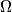$\Omega$. Let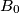$B_0$ be the midpoint of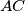$AC$ and let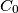$C_0$ be the midpoint of$AB$. Let$D$ be the foot of the altitude from$A$ and let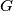$G$ be the centroid of the triangle$ABC$. Let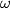$\omega$ be a circle through$B_0$ and$C_0$ that is tangent to the circle$\Omega$ at a point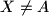$X\not= A$. Prove that the points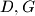$D,G$ and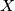$X$ are collinear.

Proposed by Ismail Isaev and Mikhail Isaev, Russia
Izvor: Međunarodna matematička olimpijada, shortlist 2011